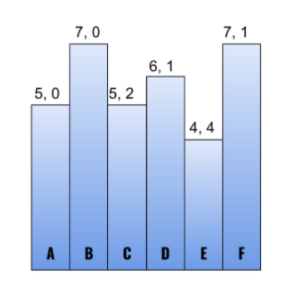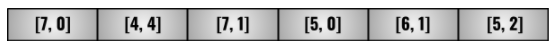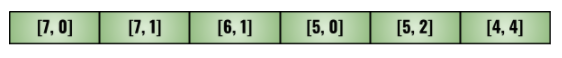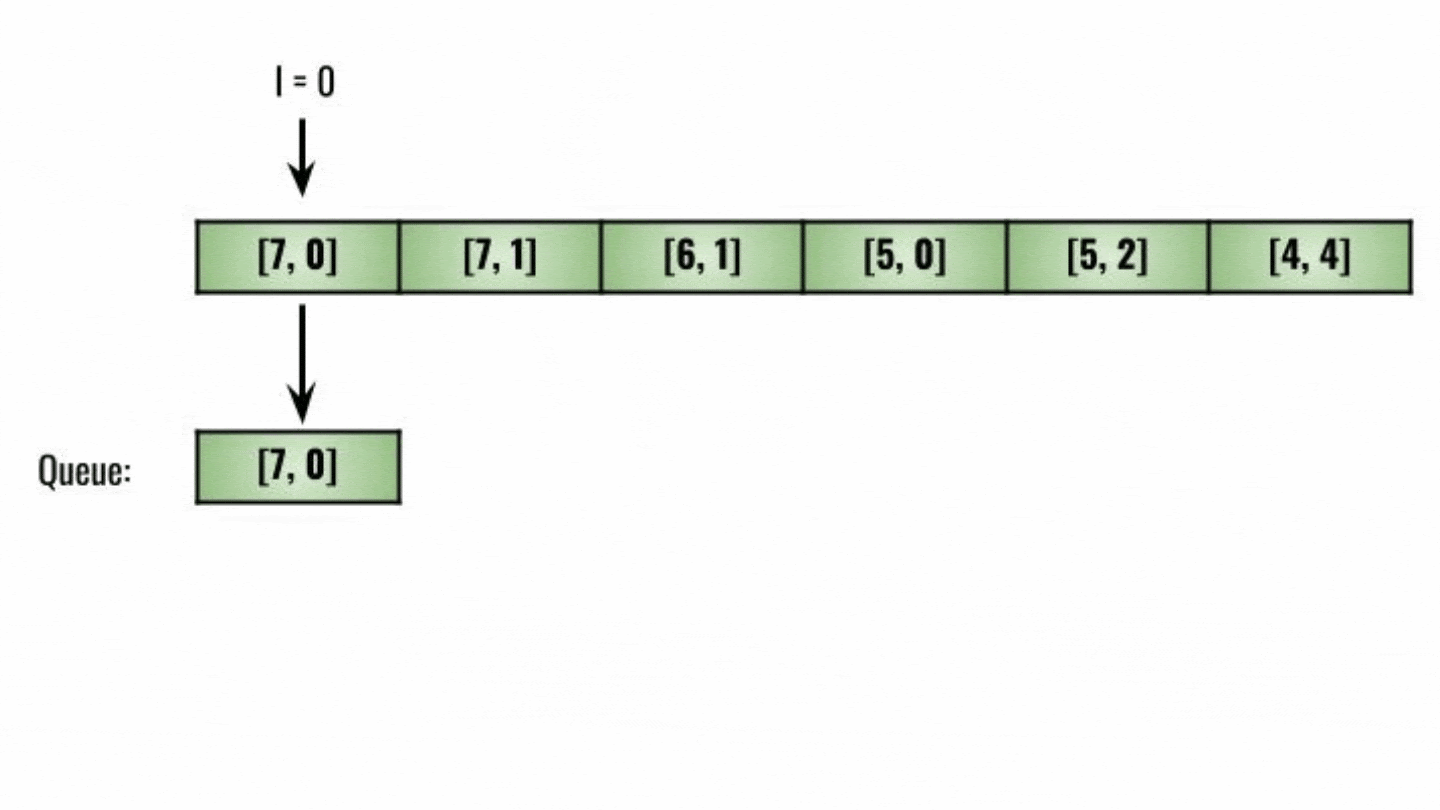# Queue Reconstruction by Height

## Problem Statement

‘N’ individuals are to be arranged in a queue. A 2-D vector ‘PEOPLE’  of size ‘N’ is given that contains the following attributes of each individual.

• Height(‘H’) of the individual.
• Number of individuals(‘K’) in the queue ahead of the current individual with greater than or equal to the height of the current individual.

Reconstruct the queue of individuals using the ‘PEOPLE’ array.

### Input

``````Enter the size of the ‘PEOPLE’ array: 6
Enter the height of each individual with the number of individuals ahead of the current individual:
7 0
4 4
7 1
5 0
6 1
5 2``````

### Output

``````Queue:
5 0
7 0
5 2
6 1
4 4
7 1``````

### Explanation

Refer to the image given below for illustration.• ‘A’ and ‘B’ have no individuals with heights greater than or equal to their respective heights.
• ‘C’ requires two individuals to be in front with heights greater than or equal to ‘C’. ‘A’ and ‘B’ fulfill the conditions.
• Similarly, requirements for other individuals are fulfilled as well and a solution to the queue reconstruction problem will be built.

## Approach

Let’s not think about ‘N’ individuals. We’ll take a series of examples to understand the procedure we need to follow for queue reconstruction.

Consider ‘A’ and ‘B’ with attributes [5,0] and [5,1], respectively. Which one of these should be in front?

Both ‘A’ and ‘B’ have equal heights. However, individual ‘B’ requires one taller or equally high individual in the front. So, we should set ‘A’ in the front and ‘B’ in the back. So, if two heights are equal, we need to choose the one in front which needs less number of individuals in front for the queue reconstruction.

Let’s consider another example with three individuals, ‘A’ and ‘B’ and ‘C’ with attributes [5,0], [7,0], and [5,2], respectively. What should be the order of individuals here?  Firstly we can set individual ‘B’ with attributes [7, 0] at index 0. The individuals in front of ‘B’ will not affect attributes of ‘B’ as it is the tallest. ‘A’ can be placed before ‘B’ as it requires no taller or equal individuals to be present before it. As no place is left, ‘C’ is set at the end. Refer to the figure for queue reconstruction given below for a better understanding.

We only need to consider people who are taller or have an equal height. Thus, for any given value of the count of taller people ahead, we should focus on the shorter people first, then the taller ones. The shorter ones wouldn't count in the taller people's fronts, and thus the order is correct.

So, in order to perform this type of iteration on our data, we must first sort it according to the criteria listed above (i.e., tallest to shortest).

Consider the example given in Problem Statement. The array of people is given as follows:Sort the data according to the heights in descending order. For equal heights, choose the individual with less number of people required in the front. So, the sorted data may look like this:Once sorting is done, we just need to insert the values on the indices equal to the number of people required in front of the current element for queue reconstruction.Refer to the algorithm given below for a better understanding of queue reconstruction problem.

## Algorithm

• Sort the ‘PEOPLE’ vector according to the comparator given below:
• If the heights of two individuals are not equal, do:
• Return the one with more height.
• Else:
• Return the one with less number of individuals required in front.
• Declare a 2-D vector ‘RESULTING_QUEUE’.
• Iterate ‘INDIVIDUAL’ from 0 to size of ‘PEOPLE’ vector, do:
• Set ‘HEIGHT’ equal to PEOPLE[INDIVIDUAL].
• Set ‘INDEX’ equal to PEOPLE[INDIVIDUAL].
• Insert HEIGHT-INDEX pair in ‘RESULTING_QUEUE’ vector at index ‘INDEX’.
• Return ‘RESULTING_QUEUE’.

## Program

``````#include <iostream>
#include <algorithm>
#include <vector>
using namespace std;

// Comparator for sorting 'PEOPLE' vector.
bool comparator(vector<int> individualA, vector<int> individualB)
{

// If the heights of two individuals are not equal.
if (individualA != individualB)
{

//Return  the one with more height.
return individualA > individualB;
}

// Else return the one with less number of individuals required in front.
return individualA < individualB;
}

vector<vector<int>> queueReconstruction(vector<vector<int>> &people)
{

// Sort the people from tallest to shortest using given comparator.
sort(people.begin(), people.end(), comparator);

// Declare a 2-D vector 'RESULTING_QUEUE'.
vector<vector<int>> resultingQueue;

for (int individual = 0; individual < people.size(); individual++)
{
int height = people[individual];
int index = people[individual];

// Insert HEIGHT-INDEX pair in 'RESULTING_QUEUE' vector at index 'INDEX'.
resultingQueue.insert(resultingQueue.begin() + index, {height, index});
}

return resultingQueue;
}

int main()
{

int n;

// Input size of the 'PEOPLE' vector.
cout << "Enter the size of the 'PEOPLE' array: ";
cin >> n;

cout << "Enter the height of each individual with the number of individuals ahead of the current individual:\n";
vector<vector<int>> people(n, vector<int>(2));

// Input every individual's attributes in 'PEOPLE' vector.
for (int i = 0; i < n; i++)
{
cin >> people[i] >> people[i];
}

cout << "Queue:\n";

// Calling QUEUE_RECONSTRUCTION() function to get the queue constructed as per the given input.
vector<vector<int>> resultingQueue = queueReconstruction(people);

// Printing the 'RESULTING_QUEUE'.
for (int individual = 0; individual < resultingQueue.size(); individual++)
{
int height = resultingQueue[individual];
int index = resultingQueue[individual];
cout << height << " " << index << endl;
}
}``````

### Input

``````Enter the size of the ‘PEOPLE’ array: 6
Enter the height of each individual with the number of individuals ahead of the current individual:
7 0
4 4
7 1
5 0
6 1
5 2``````

### Output

``````Queue:
5 0
7 0
5 2
6 1
4 4
7 1``````

## Complexity Analysis

Time Complexity: Before queue reconstruction ‘PEOPLE’ vector is sorted according to the custom criteria. Sorting a vector is an O(NlogN) operation, where ‘N’ represents the size of the vector. Insertion in a vector is a linear-time operation. For every individual in ‘PEOPLE’ from 1 to ‘N’, we are inserting the pairs in a vector. So, the time complexity of the program is O(NlogN + N2) = O(N2), where ‘N’ is the size of the input stored in ‘PEOPLE’.

Space Complexity: Additional space is required to store all the individual attributes of ‘PEOPLE’. So, space complexity is O(N).

## Key Takeaways

You have successfully learned the solution for the queue reconstruction problem. Practice and try to solve some other similar problems on our practice platform CodeStudio. If you don’t know where to start from, follow our guided paths to pave learning path. CodeStudio offers interesting blogs like this one much more. Start your learning journey today.

Happy Coding!